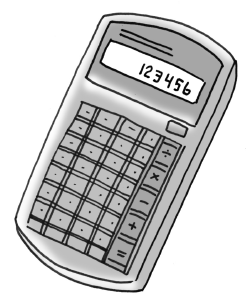### Home > CCA > Chapter AppA > Lesson A.1.7 > ProblemA-75

A-75.CALCULATOR CHECK

Use your scientific calculator to compute the value of each expression in the left-hand column below. Match each result to an answer in the right-hand column.

1. $−3 + 16 − \left(−5\right)$

1. $−16$

1. $\left(3 − 5\right)\left(6 + 2\right)$

1. $327$

1. $17\left(−23\right) + 2$

1. $0.5$

1. $5 − \left(3 − 17\right)\left(−2 + 25\right)$

1. $18$

1. $\left(−4\right)\left(−2.25\right)\left(−10\right)$

1. $−90$

1. $\frac{4-5}{-2}$

1. $−389$

Remember to be cautious when entering negative signs.
A few matches have been made below, so that you can check some of your work.

$\text{a.}\ \Rightarrow\ 4.$

$\text{f.}\ \Rightarrow\ 6.$

$\text{g.}\ \Rightarrow\ 3.$